# Data Structures in PHP## Where I Work## Slides

https://joind.in/talk/12084

http://matthewturland.com/publications

## Goals

• Learn about data structures and related terminology at a conceptual level
• Review concrete examples of concepts using PHP code
• Discuss practical applicability of concepts both within and without PHP

## SPL

The Standard PHP Library (SPL) is a collection of interfaces and classes that are meant to solve common problems.
PHP Manual

## Ardent

A Library of Collections for OO Programming

## equip/structure

Simple, immutable data structures
GitHub Repository

## spl-benchmarks

A suite of tests comparing performance of PHP SPL data structures to PHP arrays

Author: Me

## Design Patterns

A design pattern in... computer science is a formal way of documenting a solution to a design problem...
Wikipedia

## Data Structures

In computer science, a data structure is a particular way of organizing data in a computer so that it can be used efficiently.
Wikipedia

## Efficiency

• Minimal execution time
• Minimal use of storage
• With respect to code design

## Collections

In computer science, a... collection is a grouping of... data items... that have some shared significance... and need to be operated upon together...
Wikipedia

## Containers

In computer science, a container is... a data structure... used for storing objects in an organized way following specific access rules.
Wikipedia

## Arrays

In computer science, an... array is a data structure consisting of... elements... each identified by at least one array index or key...
Wikipedia

## PHP Arrays

An array in PHP is actually an ordered map... that associates values to keys... optimized for several different uses... an array, list (vector), hash table... stack, queue... trees...
PHP Manual

Semi-related note:
`array_*()` functions work only on PHP arrays

## PHP Arrays

``````
\$characters = [
'Tyrion',
'Varys',
'Arya',
'Jaqen',
];

\$author = [
'name' => 'George R. R. Martin',
'age' => 65,
'dob' => new \DateTime('1948-09-20'),
'home' => 'Sante Fe, NM',
];
``````

## SPL: `ArrayObject`

``````
\$characters = new \ArrayObject(['Melisandre']);
\$characters[] = 'Stannis';
\$characters = 'Brienne';
\$characters->append('Podrick');
\$characters->natsort();
``````

## Random Access

In computer science, random access (more precisely... direct access) is the ability to access an item of data at any given coordinates in a population of addressable elements.
Wikipedia

## Random Access

``````
\$author = [
'name' => 'George R. R. Martin',
'age' => 65,
'dob' => new \DateTime('1948-09-20'),
'home' => 'Sante Fe, NM',
];

// Random access
echo \$author['home'], \PHP_EOL;
echo \$author['name'], \PHP_EOL;

// Sequential access
foreach (\$author as \$key => \$value) {
echo \$key, ' - ', \$value, \PHP_EOL;
}
``````

## Associative Arrays

In computer science, an associative array, map, ... or dictionary is... a collection of (key, value) pairs, such that each possible key appears just once...
Wikipedia

Use case: storing an unknown number of elements for random or sequential access by unique key

## Associative Arrays

``````
\$characters = [
'name' => 'Theon',
'name' => 'Ramsay',
];
var_dump(\$characters);
``````
```array(1) {
'name' =>
string(6) "Ramsay"
}
```

## Hash Tables

In computing, a hash table (hash map) is... used to implement an associative array... A hash table uses a hash function to compute an index into an array... from which the correct value can be found.
Wikipedia

## Hash Table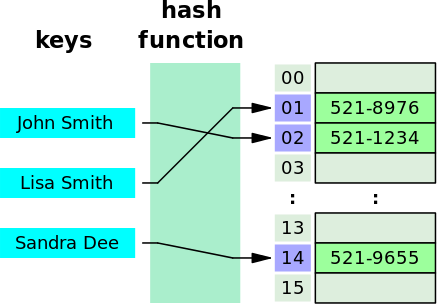``````
echo \$array['John Smith']; // 521-8976
``````

## Hash Tables in PHP

``````
echo memory_get_usage(),PHP_EOL;
\$a = [];
do {
echo memory_get_usage(),PHP_EOL;
\$a[] = count(\$a);
} while (count(\$a) < 11);
``````
`count(\$a)` `memory_get_usage()` diff
- 235600 0
0 235856 256
1 236072 216
2 236208 136
3 236344 136
...
8 237024 136
9 237224 200
10 237360 136
...

## Ardent: `Map`

``````
// equivalent to ArrayAccess::offsetGet()
function get(\$key);

// equivalent to ArrayAccess::offsetSet()
function set(\$key, \$value);

// equivalent to ArrayAccess::offsetUnset()
function remove(\$key);
``````

## Enumerated Arrays

An enumeration is a complete, ordered listing of all the items in a collection.
Wikipedia

## Enumerated Arrays in PHP

• When no array keys are specified, PHP uses consecutive integers beginning at `0` (zero)
• These are referred to as enumerated arrays
• Internally, they're no different from other (i.e. associative) PHP arrays

## Enumerated Arrays

``````
\$characters = [
0 => 'Petyr',
1 => 'Catelyn',
2 => 'Lysa',
3 => 'Sansa',
];
``````
``````
\$characters = [
0 => 'Lysa',
0 => 'Sansa',
];
var_dump(\$characters);
``````
``````
array(1) {
0 =>
string(5) "Sansa"
}
``````

## Dynamically Allocated Arrays

In computer science, ... a dynamically allocated array... is an array whose size is fixed when the array is allocated.
Wikipedia

Use case: storing a predetermined number of ordered elements for random or sequential access by position

## `SplFixedArray`

``````
\$characters = new \SplFixedArray(4);
\$characters = 'Joffrey';
\$characters = 'Robert';
\$characters = 'Drogo';
\$characters = 'Ned';
\$characters = 'Robb';
``````
``````
PHP Fatal error:  Uncaught exception 'RuntimeException'
with message 'Index invalid or out of range'
``````
``````
\$characters->setSize(5); /* Avoid doing this */
\$characters = 'Robb';
``````

## Vectors

In computer science... a one-dimensional array.
Wikipedia

## Ardent: `Vector`

``````
function appendAll(\Traversable \$traversable);
function append(\$item);
function removeItem(\$object);
function apply(callable \$callable);
function map(callable \$map): Vector;
function reduce(\$initialValue, callable \$combine): Vector;
function filter(callable \$filter): Vector;
function limit(int \$n): Vector;
function skip(int \$n): Vector;
function slice(int \$start, int \$count): Vector;
function keys(): Vector;
``````

In computer science, a linked list is a data structure consisting of a group of nodes which together represent a sequence.
Wikipedia

Use case: storing and accessing an unknown number of ordered elements sequentially

## Linked Lists vs Hash Tables

• Pro: dynamic size without hash function overhead
• Con: random access is less efficient
• Con: random insertion is less efficient## SPL: `SplDoublyLinkedList`

``````
function unshift(\$value);
function push(\$value);

function shift();
function pop();

function top();
function bottom();
``````

## SPL: `SplDoublyLinkedList`

• Favor iteration over random access
• Add: use `unshift()` or `push()` vs `add()`
• Remove: use `shift()` or `pop()`
• Access: use `top()` or `bottom()`

## Ardent: `LinkedList`

``````
function unshift(\$value);
function push(\$value);
function insertBefore(int \$position, \$newval);
function insertAfter(int \$position, \$newval);

function shift();
function pop();

function first();
function last();
function seek(int \$position);

function indexOf(\$value, callable \$f = null): int;
function contains(\$value, callable \$f = null): bool;
``````

## Ardent: `LinkedList`

• Favor iteration over random access
• Add: use `unshift()` or `push()` vs `insertBefore()` / `insertAfter()`
• Remove: use `shift()` or `post()`
• Access: use `first()` or `last()` vs `seek()`
• Avoid using `indexOf()` or `contains()`

## Stacks

Credit: @ninjagrl## Stacks

In computer science, a stack... is... a collection of elements, with two principal operations: 1) push adds an element to the collection; 2) pop removes the last element that was added.
Wikipedia

Use case: storing an unknown number of elements where only the latest element stored is accessible

## Using Stacks

• Stacks are basically linked lists where elements are only added and removed from the front, called the top of the stack
• Useful for avoiding recursion in situations that require backtracking, such as parsing and searching / traversing trees -- more on those later

## SPL: `SplStack`

• Extends `SplDoublyLinkedList`
• `setIteratorMode()` provides support for removing elements or not during iteration
• Limited to LIFO iteration order

## SPL: `SplStack`

``````
\$stack = new \SplStack;
\$stack->push('Cersei');
\$stack->push('Jamie');
\$stack->push('Tywin');
var_dump(\$stack->pop());
var_dump(\$stack->pop());
var_dump(\$stack->bottom());
var_dump(\$stack->pop());
``````
``````
string(5) "Tywin"
string(5) "Jamie"
string(6) "Cersei"
string(6) "Cersei"
``````

## Ardent: `LinkedStack`

``````
\$stack->push('Cersei');
\$stack->push('Jamie');
\$stack->push('Tywin');
var_dump(\$stack->pop());
var_dump(\$stack->pop());
var_dump(\$stack->last());
var_dump(\$stack->pop());
``````
``````
string(5) "Tywin"
string(5) "Jamie"
string(6) "Cersei"
string(6) "Cersei"
``````

## Queues

In computer science, a queue is a... collection in which the... operations... are the addition of entities to the rear terminal position, known as enqueue, and removal of entities from the front terminal position, known as dequeue.
Wikipedia

Use case: storing an unknown number of elements where only the element added earliest is accessible

## Using Queues

• Queues are basically linked lists where elements are only added to the back of the list (enqueue) and removed from the front of the list (dequeue)
• Useful for prioritization or scheduling of tasks based on the order in which they're added

## Queueing Theory

Queueing theory is the... study of waiting lines, or queues... so that queue lengths and waiting time can be predicted.
Wikipedia

## Queueing Theory Illustrated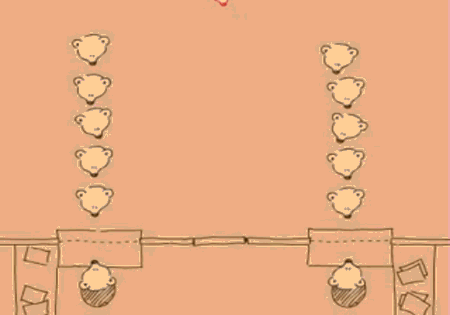## SPL: `SplQueue`

• Extends `SplDoublyLinkedList`
• `setIteratorMode()` provides support for removing elements or not during iteration
• Limited to FIFO iteration order
• `enqueue()` and `dequeue()` alias `push()` and `shift()` respectively

## SPL: `SplQueue`

``````
\$queue = new \SplQueue;
\$queue->enqueue('Viserys');
\$queue->enqueue('Daenerys');
\$queue->enqueue('Jon');
var_dump(\$queue->dequeue());
var_dump(\$queue->dequeue());
var_dump(\$queue->top());
var_dump(\$queue->dequeue());
``````
``````
string(7) "Viserys"
string(8) "Daenerys"
string(3) "Jon"
string(3) "Jon"
``````

## Ardent: `LinkedQueue`

``````
\$queue->enqueue('Jorah');
\$queue->enqueue('Barristan');
\$queue->enqueue('Daario');
var_dump(\$queue->dequeue());
var_dump(\$queue->dequeue());
var_dump(\$queue->first());
var_dump(\$queue->dequeue());
``````
``````
string(5) "Jorah"
string(9) "Barristan"
string(6) "Daario"
string(6) "Daario"
``````

## Priority Queues

In computer science, a priority queue is... like a regular queue... but where... each element has a "priority"... an element with high priority is served before an element with low priority.
Wikipedia

Use case: storing an unknown number of elements with associated priorities where only the highest priority most recently added element is accessible

## SPL: `SplPriorityQueue`

``````
\$queue = new \SplPriorityQueue;
\$queue->insert('value1', 'priority0');
\$queue->insert('value2', 'priority1');
\$queue->insert('value0', 'priority2');
var_dump(\$queue->extract());
var_dump(\$queue->extract());
var_dump(\$queue->extract());
``````
``````
string(6) "value0"
string(6) "value2"
string(6) "value1"
``````

## Priority Queues in the Wild

• Job queues implement this structure
• Check out Bernard

## Graphs

In computer science, a graph is... a finite... set of nodes or vertices, together with... pairs of these nodes... known as edges.
Wikipedia

No SPL or Ardent implementations; see graphp

Use case: modeling relationships between objects

## Graph Theory

In mathematics and computer science, graph theory is the study of graphs... to model pairwise relations between objects.
Wikipedia

## Directed Graphs

In... graph theory, a directed graph (or digraph) is a graph... where the edges have a direction associated with them.
Wikipedia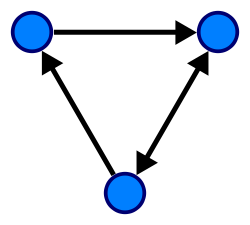## Cycle Graph

In graph theory, a cycle graph or circular graph is a graph that consists of... some number of vertices connected in a closed chain.
Wikipedia## graphp

``````
{
"require": {
"graphp/graph": "^0.7"
}
}
``````
``````
use Fhaculty\Graph\Graph;
\$graph = new Graph;
\$rome = \$graph->createVertex('Rome');
\$cologne = \$graph->createVertex('Cologne');
\$rome->createEdgeTo(\$rome);
foreach (\$rome->getVerticesEdgeFrom() as \$vertex) {
echo \$vertex->getId(), ' leads to Rome', \PHP_EOL;
}
``````
``````
``````

## Trees

In... graph theory, a tree is an undirected graph in which any two vertices are connected by exactly one path... without... cycles...
Wikipedia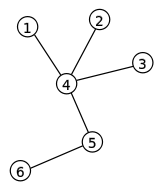## Trees

In computer science, a tree is a... data structure... with a root value and subtrees of children...
Wikipedia## Trees in PHP

• If you've used the DOM or SimpleXML extensions, you've used tree-based XML parsers
• SPL has no implementations, but Ardent does...

## Binary Trees

In computer science, a binary tree is a tree... in which each node has at most two children.
Wikipedia

## Ardent: `BinaryTree`

Tree example from earlier:

``````
use Collections\BinaryTree as T;
\$nine = new T(9);
\$nine->setLeft(new T(4));
\$five = new T(5);
\$five->setRight(\$nine);
\$two = new T(2);
\$two->setRight(\$five);
\$eleven = new T(11);
\$six = new T(6);
\$eleven->setLeft(new T(5));
\$eleven->setRight(new T(11));
\$seven = new T(7);
\$seven->setLeft(new T(2));
\$seven->setRight(\$six);
``````

## Binary Search Trees

In computer science, binary search trees (BST)... store data items, known as keys, and allow fast insertion and deletion of such keys, as well as checking whether a key is present in a tree.
Wikipedia

## AVL Trees

In computer science, an AVL tree... is a self-balancing binary search tree.
Wikipedia## Ardent: `AvlTree`

Implements `Countable` and `IteratorAggregate`

``````
function __construct(callable \$comparator = null);
function remove(\$element);
function contains(\$item): bool;
``````

## Heaps

In computer science, a heap is a specialized tree-based data structure that satisfies the heap property: if A is a parent node of B then... A is ordered with respect to... B...
Wikipedia

Use case: storing an unknown number of elements ordered based on a comparator function as applied to those elements

## Using Heaps

Useful for sorting elements
with minimal reordering as more are added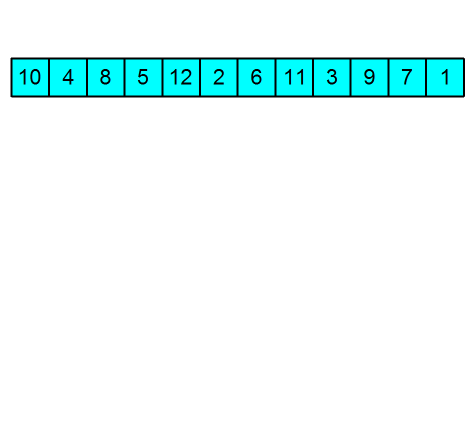## SPL: `SplHeap`

• Abstract class that implements a heap but requires a comparator function, `compare()`, to be defined by subclasses
• Implements `Countable` and `Iterator`, where iteration results in element extraction

## SPL: `SplMinHeap`

``````
\$heap = new \SplMinHeap;
\$heap->insert(3);
\$heap->insert(2);
\$heap->insert(1);
foreach (\$heap as \$n) {
var_dump(\$n);
}
var_dump(count(\$heap));
``````
``````
int(1)
int(2)
int(3)
int(0)
``````

## SPL: `SplMaxHeap`

``````
\$heap = new \SplMaxHeap;
\$heap->insert(1);
\$heap->insert(2);
\$heap->insert(3);
foreach (\$heap as \$n) {
var_dump(\$n);
}
var_dump(count(\$heap));
``````
``````
int(3)
int(2)
int(1)
int(0)
``````

## Sets

In computer science, a set... can store certain values, without any particular order, and no repeated values... rather than retrieving a specific element... one typically tests a value for membership...
Wikipedia

## Set Theory

Set theory is the branch of mathematical logic that studies sets.
Wikipedia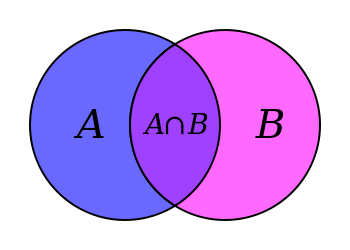## Set Operations

Union
all unique items across multiple sets
Intersection
items common to multiple sets
Difference
items not common to multiple sets
Complement
items contained in one set and not others
Cartesian Product
all possible pairings of items from two sets

## SPL: `SplObjectStorage`

• Use `attach()` and `detach()` to add and remove elements
• `addAll()`, `removeAll()`, and `removeAllExcept()` (in PHP >= 5.3.6) perform the union, complement, and intersection set operations respectively

## Typecasting for `SplObjectStorage`

``````
\$bran = (object) 'Bran';
\$hodor = (object) 'Hodor';
\$osha = (object) 'Osha';
\$a = new \SplObjectStorage;
\$a->attach(\$bran);
\$a->attach(\$hodor);
\$b = new \SplObjectStorage;
\$b->attach(\$hodor);
\$b->attach(\$osha);
``````

## Union with `SplObjectStorage`

``````
/* \$a = ['Bran', 'Hodor']; \$b = ['Hodor', 'Osha']; */
\$union = clone \$a;
echo implode(PHP_EOL, array_map(
function(\$o) { return \$o->scalar; },
iterator_to_array(\$union)
));
``````
``````
Bran
Hodor
Osha
``````

## Complement with `SplObjectStorage`

``````
/* \$a = ['Bran', 'Hodor']; \$b = ['Hodor', 'Osha']; */
\$complement = clone \$a;
\$complement->removeAll(\$b)

echo implode(PHP_EOL, array_map(
function(\$o) { return \$o->scalar; },
iterator_to_array(\$complement)
));
``````
``````
Bran
``````

## Intersection with `SplObjectStorage`

``````
/* \$a = ['Bran', 'Hodor']; \$b = ['Hodor', 'Osha']; */
\$intersection = clone \$a;
\$intersection->removeAllExcept(\$b);

echo implode(PHP_EOL, array_map(
function(\$o) { return \$o->scalar; },
iterator_to_array(\$intersection)
));
``````
``````
Hodor
``````

## Difference with `SplObjectStorage`

``````
/* \$a = ['Bran', 'Hodor']; \$b = ['Hodor', 'Osha']; */
\$intersection = clone \$a;
\$intersection->removeAllExcept(\$b);

\$union = clone \$a;

\$difference = clone \$union;
\$difference->removeAll(\$intersection);

echo implode(PHP_EOL, array_map(
function(\$o) { return \$o->scalar; },
iterator_to_array(\$difference)
));
``````
``````
Bran
Osha
``````

## Ardent: `Set`

``````
function has(\$item): bool;
function remove(\$item);
function difference(Set \$that): Set;
function intersection(Set \$that): Set;
function complement(Set \$that): Set;
function union(Set \$that): Set;
``````

## Sets in the Wild

• SQL `JOIN`s are somewhat analogous to a limited Cartesian product of two sets
• Some SQL dialects also support the union, intersection, and difference set operations